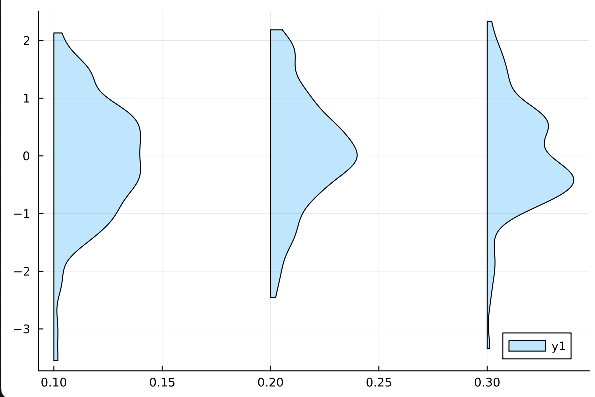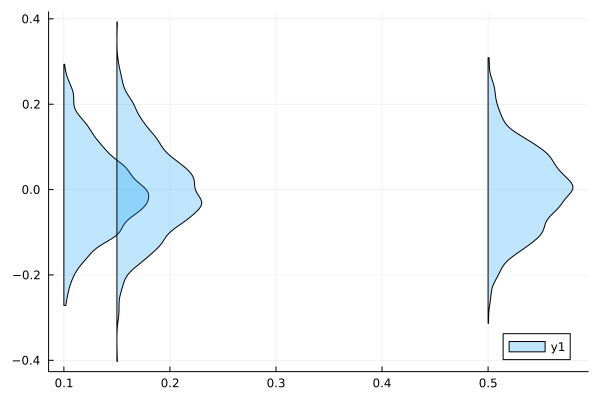# How to scale the density of violin plot

I would like to use a violin plot to display density information. For the x-axis, the scaling matters. However, only the shape of the densities matter. How can I rescale the densities to prevent occlusion?

Here is a simple example:

``````using StatsPlots
violin(repeat([.1,.2,.3],outer=100),randn(300), side=:right)
``````

Thanks!

It seems to help to use `groupedviolin` instead

``````groupedviolin(repeat([.1,.2,.3],outer=100),randn(300), side=:right, fillalpha=0.25)
``````1 Like

Thank you. That does help quite a bit in many cases. It does break down when the spacing is variable:

`groupedviolin(repeat([.1,.15,.5],outer=100),randn(3000)*.1, side=:right, fillalpha=0.25)`I noticed the keyword `spacing` in the linked function, but it did not seem to have an effect on the space.

Somewhat related question: is it possible to use fillalpha between quantiles, such as at .025 and .975?